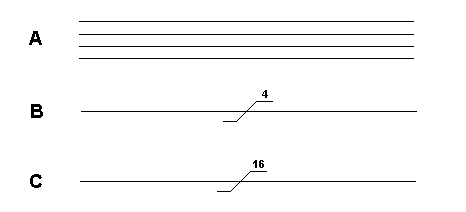Home > Electronic Tutorials > Microprocessor Systems Tutorial > Buses Tutorial

Microprocessor Systems - Electronic Tutorials

Buses TutorialA bus is a collection of wires. Diagram A is a four bit bus. Zeros and ones can be put on the bus, 0 volts for zero and +5 volts to represent a one. The smallest number that can be put on a four bit bus is 0000. The largest is 1111 which is 15 in decimal and F in hex. Therefore sixteen different numbers can be placed on this bus, 0000 being the lowest and 1111 the highest. Rather than draw four wires, we use the representation shown in diagram B.

Many microprocessor systems use a eight bit data bus. The smallest number that can be placed on it is 00000000. The largest number is 11111111 which is equivalent to 255 in decimal. Therefore 256 different numbers can be placed on this bus. 255 in decimal is FF in hex.

A sixteen bit bus is shown in diagram C. The smallest number we can put on this bus is 0000000000000000. The largest number is 1111111111111111 which is 65535 in decimal. Therefore 65536 different numbers can be put on this bus. 65535 in decimal is FFFF in hex.

All the registers in the memory chips have their own individual addresses, like house numbers in a street. By putting its address, in binary, on an address bus we can select any individual register. Address buses are commonly 16 bits, so we can select any one of 65536 registers.